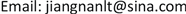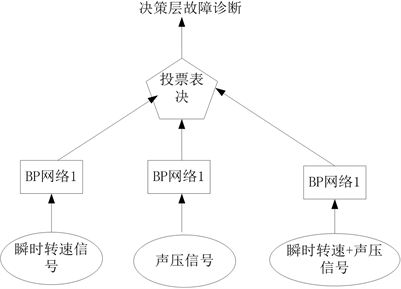﻿ 基于组合分类器的MSF发动机综合故障诊断研究 Study on the Comprehensive Fault Diagnosis about MSF Engine Based on the Combining Classifier

Operations Research and Fuzziology
Vol. 09  No. 02 ( 2019 ), Article ID: 30536 , 7 pages
10.12677/ORF.2019.92020

Study on the Comprehensive Fault Diagnosis about MSF Engine Based on the Combining Classifier

Tao Li*, Li Chen, Song Gao

Security Department of Air Ammunition Air Force Service College, Xuzhou JiangsuReceived: May 9th, 2019; accepted: May 23rd, 2019; published: May 30th, 2019ABSTRACT

Aiming at the engine characteristics of fault location trouble and large amount of information in instantaneous speed and pressure, the fusion algorithm of the decision level about the combining classifiers is proposed based on voting by ballot; the deficiency of neural network learning algorithm is pointed out; and the learning algorithm is improved; with voting scheme using three BP network, integrated fault diagnosis method of engine combined MSF is proposed based on neural network classifier. Through the experimental research and comparative analysis, the method is more reliable and effective in improving the comprehensive fault diagnosis capability of the engine.

Keywords:Vote by Ballot, Combining Classifier, Neural Network, Fault Diagnosis1. 引言

2. 多传感器信息集成与融合MSF的基本原理

3. 基于投票表决方案的组合分类器决策层信息融合算法

${T}_{k}\left(x\in {C}_{i}\right)=\left\{\begin{array}{cc}1& {e}_{k}\left(x\right)=i,i\in \Lambda \\ 0& 其它值\end{array}$ (1)

$E\left(x\right)=\left\{\begin{array}{ccc}j& 如果& \exists j\in \Lambda ,{\cap }_{k=1}^{k}{T}_{k}\left(x\in {C}_{j}\right)>0\\ M+1& & 其它值\end{array}$ (2)

$E\left(x\right)=\left\{\begin{array}{ccc}j& 如果& \exists j\in \Lambda ,{\cap }_{k=1}^{k}\left\{{T}_{k}\left(x\in {C}_{j}\right)\cup \left(1-{\cup }_{q=1}^{M}{T}_{k}\left(x\in {C}_{q}\right)\right)\right\}>0\\ M+1& & 其它值\end{array}$ (3)

$E\left(x\right)=\left\{\begin{array}{ccc}j& 如果& {T}_{E}\left(x\in {C}_{i}\right)={\mathrm{max}}_{i\in \Lambda }{T}_{E}\left(x\in {C}_{i}\right)>\frac{K}{2}\\ M+1& & 其它值\end{array}$ (4)

$E\left(x\right)=\left\{\begin{array}{ccc}j& 如果& {T}_{E}\left(x\in {C}_{i}\right)={\mathrm{max}}_{i\in \Lambda }{T}_{E}\left(x\in {C}_{i}\right)>\alpha \cdot K\\ M+1& & 其它值\end{array}$ (6)

${\chi }_{1}={\mathrm{max}}_{i\in \Lambda }{T}_{E}\left(x\in {C}_{i}\right)$ (7)

${\chi }_{2}={\mathrm{max}}_{i\in \Lambda -\left\{j\right\}}{T}_{E}\left(x\in {C}_{i}\right)$ (8)

$E\left(x\right)=\left\{\begin{array}{ccc}j& 如果& {T}_{E}\left(x\in {C}_{j}\right)={\chi }_{1}并且\left({\chi }_{1}-{\chi }_{2}\right)>\beta \cdot K\\ M+1& & 其它值\end{array}$ (9)

4. BP神经网络学习算法的缺点分析及改进方法

4.1. BP学习算法的缺点分析

BP网络的误差公式为

$E=\frac{1}{2}\underset{p}{\sum }\underset{k}{\sum }{\left({t}_{pk}-{O}_{pk}\right)}^{2}$ (10)

${O}_{pk}=f\left(ne{t}_{k}\right)$ 是一个非线性函数，而多层的BP网络中 $ne{t}_{k}$ 又是前一层神经元的非线性函数，用 $\epsilon$ 表示其中一个样本 ${P}_{1}$ 的误差，则

$\epsilon \left(W,{t}_{{p}_{1}},{X}_{{p}_{1}}\right),{E}_{{p}_{1}}=\frac{1}{2}\underset{{p}_{1}}{\sum }\epsilon \left(W,{t}_{{p}_{1}},{X}_{{p}_{1}}\right)$ (11)

${E}_{p1}$ 与权 $W$ 有关，与输入的学习样本和输出样本有关，如暂且不考虑样本的问题， ${E}_{p1}$ 是一个与权矢量相关的函数，在多层BP网络中，权空间的维数 ${n}_{w}$ 为： ${n}_{w}=ij+ik$ ，在 ${n}_{w}+1$ 维的空间中，误差 ${E}_{p1}$ 是一个具有极其复杂形状的曲面，如果再考虑输入的样本，则 ${E}_{p1}$ 的形状就更难想象  。对这样的梯度，其结果不像线性阈值单元的网络那么简单，对于BP网络的误差曲面，有如下三点缺点：第一，有很多全局最小的解；存在一些平坦区，在此区内误差改变很小，这些平坦区多数发生在神经元的输出接近于0或1的情况下，对于不同的映射；其平坦区的位置、范围各不相同，有的情况下，误差曲面会出现一些阶梯状。第三，存在不少局部最小点，在某些初始值的条件下，算法的结果会陷入局部最小，使算法不收敛  。针对BP算法的缺点，采取如下措施：

4.2. 基于动量项和变步长的BP学习算法

${\Delta }_{p}{W}_{ji}\left(t+1\right)=\eta {\delta }_{pj}\cdot {O}_{pi}+\alpha {\Delta }_{p}{W}_{ji}\left(t\right)$ (12)

BP算法存在的问题之一是 $\eta$$\alpha$ 是由经验确定的。显然，对于学习率 $\eta$ 而言， $\eta$ 越大，权重变化越大，收敛越快，但是 $\eta$ 越大也容易引起振荡。因此 $\eta$ 应按照这样的原理选取：在不致引起振荡的情况下，尽可能取越大的 $\eta$

(1) 设置权值 ${W}_{jt}$ 和阈值 ${\theta }_{j}$ 的初始值为一小的随机数，给定允许误差 $\epsilon <0$ (很小的正数)；

(2) 提供训练样本，输入向量 ${X}_{p}$ 和输出向量 ${d}_{p}\left(p=1,2,\cdots ,P\right)$ ，对每个 $p$ 进行(3)到(5)；

(3) 计算实际输出及隐层单元的状态；

${O}_{Pj}={f}_{j}\left(ne{t}_{pj}\right)={f}_{j}\left(\underset{i}{\sum }{W}_{ji}{O}_{i}\right)$ (13)

(4) 计算训练误差；

${\delta }_{pj}=\left({t}_{pj}-{O}_{pj}\right){O}_{pj}\left(1-{O}_{pj}\right)$ (输出层) (14)

${\delta }_{pj}={O}_{pj}\left(1-{O}_{pj}\right)\underset{K}{\sum }{\delta }_{PK}{W}_{Kj}$ (隐层) (15)

(5) 修正权值或阈值；

${W}_{ji}\left(t+1\right)={W}_{ji}\left(t\right)+\eta {\delta }_{pj}{O}_{pi}+\alpha {W}_{ji}\left(t\right)-{W}_{ji}\left(t-1\right)$ (16)

${\theta }_{j}\left(t+1\right)={\theta }_{j}\left(t\right)+\eta {\delta }_{pj}+\alpha \left({\theta }_{j}\left(t\right)-{\theta }_{j}\left(t-1\right)\right)$ (17)

(6) 当 $p$ 历经1到 $P$ 后，判断训练指标是否满足要求，若 $E\left(t\right)<\epsilon$ ，则转(8)，否则转(7)；

(7) 若 $E\left(t\right) 则转(2)，使 $p$ 循环1到 $P$ ；若 $E\left(t\right)\ge E\left(t-1\right)$ ，则 $\eta =\eta /2$ 转(2)，使 $p$ 循环1到 $P$

(8) 停止。

4.3. 修改作用函数

$f\left(x\right)=1/\left(1+{e}^{-ax}\right)$ (18)

${\delta }_{Pk}=\alpha \left({t}_{pk}-{O}_{pk}\left(1-{O}_{pK}\right)\right)$ (19)

${\delta }_{Kj}=\alpha {O}_{Kj}\left(1-{O}_{Pj}\right)\underset{K}{\sum }\left({\delta }_{PK}{W}_{Kj}\right)$ (20)Figure 1. Curve: system result of standard experiment

5. 基于组合分类器的MSF发动机综合性故障诊断应用Figure 2. Curve: system result of standard experiment

$识别率=\frac{正确分类的样本数}{总样本数}×100%$ (21)Table 1. System resulting data of standard experiment

6. 结论

Study on the Comprehensive Fault Diagnosis about MSF Engine Based on the Combining Classifier[J]. 运筹与模糊学, 2019, 09(02): 170-176. https://doi.org/10.12677/ORF.2019.92020

1. 1. 李岳. 基于智能诊断方法的摩托车发动机故障检测系统研究[J]. 国防科技大学学报, 1997, 19(3): 84-88.

2. 2. 边肇祺. 模式识别[M]. 北京: 清华大学出版社, 1986.

3. 3. 殷勤业, 杨宗凯, 谈兵, 等. 模式识别与神经网络[M]. 北京: 机械工业出版社, 1992.

4. 4. 焦李成, 等. 神经网络系统理论[M]. 西安: 西安电子科技大学出版社, 1996.

5. 5. 廖贵敏, 等. 基于参数漂移量统计分析的电路可靠度预计[C]//第十五届电路与系统学术年会NSCAS. 广州, 1999: 1021-1024.

6. 6. 闻新, 张洪钺, 周露. 控制系统的故障诊断和容错控制[M]. 北京: 机械工业出版社, 1998.

7. 7. 曾天翔, 等. 电子设备测试性及诊断技术[M]. 北京: 航空工业出版社, 1995.

8. 8. 范志锋, 崔平, 文健, 吕静. 基于退化敏感参数的弹药控制系统储存寿命评估[J]. 弹箭与制导学报, 2017, 33(5): 109-111.

9. 9. 田中大, 高宪文, 李琨. 基于KPCA与LSSVM的网络控制系统时延预测方法[J]. 系统工程与电子技术, 2013, 35(6): 1281-1285.

10. 10. 李玲玲, 马东娟, 李志刚. 基于状态监测数据的电器电接触性能评估[J]. 机械工程学报, 2015, 51(9): 198-203.

11. 11. 沙起才, 编译. 航空地面装备配备量模型试验[J]. 外国空军后勤, 2005(4).

12. 12. 吕永波, 彭宏勤. 铁路运输系统劳动力合理配备模型的研究[J]. 北方交通大学学报, 1997, 21(3): 326-330.

13. 13. 宋太亮. 装备保障性工程[M]. 北京: 国防工业出版社, 2012.

14. 14. 程海彬, 江云, 鲁浩, 等. 基于DGPS的弹载捷联惯导系统性能评估技术研究[J]. 航空兵器, 2015(3): 23-26.

15. NOTES

*第一作者。